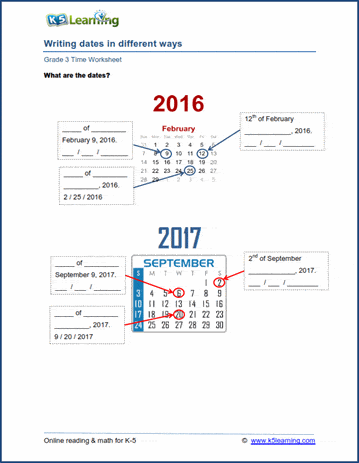# 3rd Grade Math Worksheets: Telling Time

## Time and calendar worksheets for grade 3

Our grade 3 time worksheets give students additional practice in reading an analog clock face, as well as calculating intervals of time, estimating time, converting units of time and working with calendars (reading and writing dates, elapsed time on a calendar, full year calendars).

## Time phrases

Time phrases worksheet Half past 12   -  12:30

## Telling time worksheets (analog clocks)

Telling time (5 minute intervals)

Draw the clock (5 min intervals)

Telling time (1 min intervals)

Draw the clock (1 min intervals)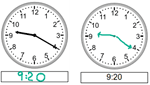## Elapsed time

How much time went by?

What time was it / will it be (15 min intervals)

What time was it / will it be (5 min intervals)

What time was it / will it be (1 min intervals)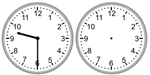## Estimating & rounding time

Estimating time - how long various activities take Sing a song  -  3 minutes
Rounding time worksheets  (to nearest hour, quarter hour, 5 min)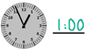## Converting units of time

Converting units of time (sec, min, hours, days, weeks, years) 3 hours =  ____ minutes

## Calendars

Months as ordinal numbers June = 6th month
Writing Dates June 6, 2020

Elapsed time on a calendar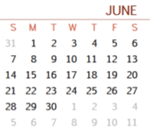## Time word problems

Grade 3 time word problems Word problems